# The MI Procedure

### EM Algorithm for Data with Missing Values

The EM algorithm (Dempster, Laird, and Rubin, 1977) is a technique that finds maximum likelihood estimates in parametric models for incomplete data. For a detailed description and applications of the EM algorithm, see the books by Little and Rubin (2002); Schafer (1997); McLachlan and Krishnan (1997).

The EM algorithm is an iterative procedure that finds the MLE of the parameter vector by repeating the following steps:

1. The expectation E-step Given a set of parameter estimates, such as a mean vector and covariance matrix for a multivariate normal distribution, the E-step calculates the conditional expectation of the complete-data log likelihood given the observed data and the parameter estimates.

2. The maximization M-step Given a complete-data log likelihood, the M-step finds the parameter estimates to maximize the complete-data log likelihood from the E-step.

The two steps are iterated until the iterations converge.

In the EM process, the observed-data log likelihood is nondecreasing at each iteration. For multivariate normal data, suppose there are G groups with distinct missing patterns. Then the observed-data log likelihood being maximized can be expressed aswhere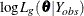is the observed-data log likelihood from the gth group, and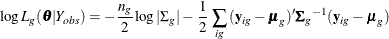where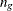is the number of observations in the gth group, the summation is over observations in the gth group,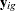is a vector of observed values corresponding to observed variables,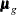is the corresponding mean vector, and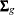is the associated covariance matrix.

A sample covariance matrix is computed at each step of the EM algorithm. If the covariance matrix is singular, the linearly dependent variables for the observed data are excluded from the likelihood function. That is, for each observation with linear dependency among its observed variables, the dependent variables are excluded from the likelihood function. Note that this can result in an unexpected change in the likelihood between iterations prior to the final convergence.

See Schafer (1997, pp. 163–181) for a detailed description of the EM algorithm for multivariate normal data.

By default, PROC MI uses the means and standard deviations from available cases as the initial estimates for the EM algorithm. The correlations are set to zero. These estimates provide a good starting value with positive definite covariance matrix. For a discussion of suggested starting values for the algorithm, see Schafer (1997, p. 169).

You can specify the convergence criterion with the CONVERGE= option in the EM statement. The iterations are considered to have converged when the maximum change in the parameter estimates between iteration steps is less than the value specified. You can also specify the maximum number of iterations used in the EM algorithm with the MAXITER= option.

The MI procedure displays tables of the initial parameter estimates used to begin the EM process and the MLE parameter estimates derived from EM. You can also display the EM iteration history with the ITPRINT option. PROC MI lists the iteration number, the likelihood –2 log L, and the parameter valuesat each iteration. You can also save the MLE derived from the EM algorithm in a SAS data set by specifying the OUTEM= option.